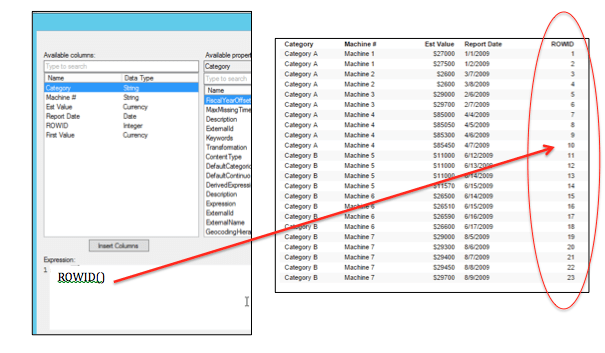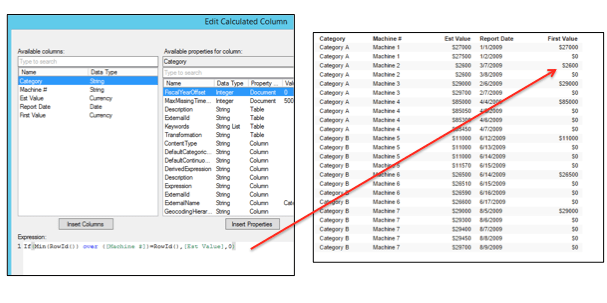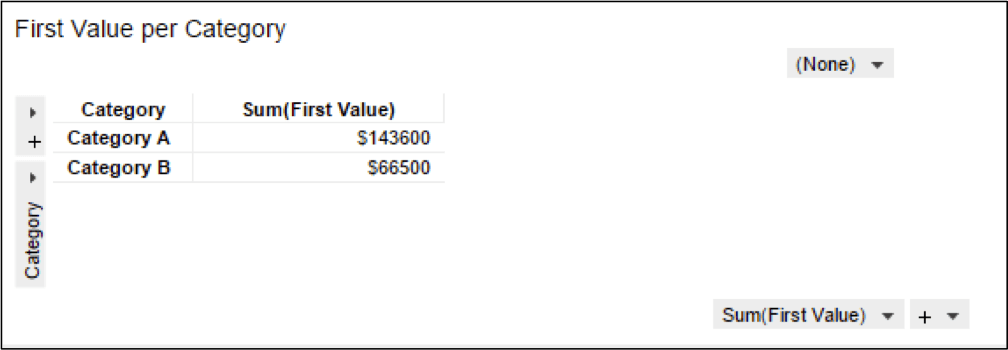# Solving a Common Problem Using the Spotfire ROWID Function

Problem description:

I’ve worked on a number of projects lately where a common problem has been presented. The scenario goes something like this:

Consider the Spotfire table below. For each category, there are several machines. Daily estimated values for each machine are reported over a period of time. When I add up the total value of all machines for each category, I only want to SUM the first reported value for each machine. All other values need to be ignored. The difficulty in accomplishing this task is that it requires access to the individual cells in the table. Fortunately, there is a solution! Enter the “ROWID” function.

The solution to this and many other problems where accessing unique values in individual cells is required, is to make use of the “ROWID( )” function. This function simply assigns a row number to each row in the data table. Once this assignment has been made, you can reference individual rows using the “ROWID” value that has been assigned.

For example: If I was to simply insert a new calculated column using the ROWID function this is what I would see. Each row is assigned a unique row number.Let’s use the ROWID function to accomplish the task outlined above.   To do this, we will create a new calculated column that does the following:

1.Determine which row ID is the first-row ID for each machine, by calculating the smallest “ROWID” value for each machine. The logic is shown below.

Min(RowID( ) ) over( [ Machine # ] )

2. If the current row being evaluated is the first row for each machine, as determined by the logic above, then assign the table value. Otherwise, assign a value of zero.

If(Min(RowId()) over ([Machine #])=RowId(),[Est Value],0)

3. Use the function above to insert a new calculated column that we will name “First Value”. Notice that the new column contains a value for only the first report date for each machine.4. Finally, we use a cross-tab table to SUM all of the values in our new column for each category.Summary: Understanding how the ROWID( ) function works can be a valuable tool when the task requires access to individual rows in a Spotfire Data Table. The example above illustrates how to sum only the first listed value of a secondary category. However, the ROWID( ) function can also be used in a wide variety of other use cases.

Keep up with all of the other Spotfire Tips and Tricks posts, right here on The TIBCO Blog.